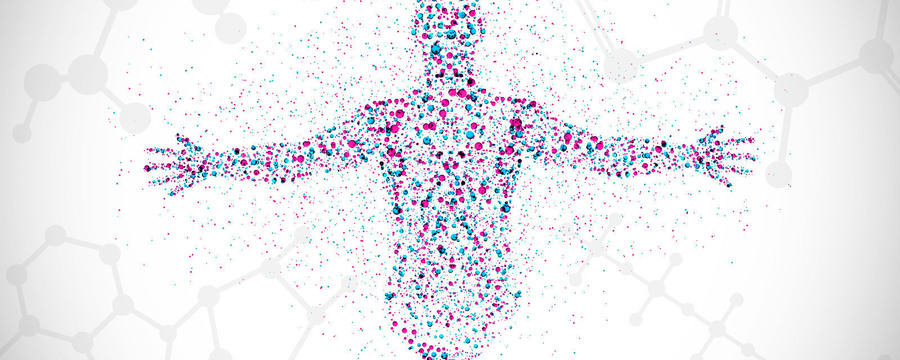﻿ Introduction to the application of univariate data analysis in metabolomics
•# Introduction to the application of univariate data analysis in metabolomics

An introduction to univariate data analysis approaches used in metabolomics.
7.8
JASPER ENGEL: Univariate data analysis approaches are popular tools in biostatistical analysis to determine if the values that were measured for a specific variable differ significantly between groups of samples. This variable may correspond to a specific peak in a mass spectrum or the concentration of a specific metabolite. Since univariate methods only analyse one variable at a time, they essentially assume that the metabolites are operating independently from each other. Clearly, this assumption is not correct, since metabolites are related to each other via complex networks. Because of this, subtle metabolic differences may not be detected. Such differences can be detected by multivariate methods that take metabolite relationships into account.
50.1
However, these methods can be difficult to apply to metabolomics data, especially when the number of variables in a data set is much larger than the number of samples. Therefore, univariate and multivariate approaches are complementary to each other and are both important approaches in the metabolomics toolbox. There are essentially two types of univariate methods. You have so-called parametric methods that assume that the data has come from specific probability distribution, which is often assumed to be a normal distribution. Non-parametric approaches make no such assumptions. Generally speaking, parametric models make more assumptions about the data and can therefore produce better results when these assumptions are correct. However, their results can also be very misleading when the assumptions are incorrect.
99.4
If it is assumed that the data follow a normal distribution, the two-sample t-test may be applied to compare two groups of samples to each other. A one-way Analysis of Variance, or ANOVA, can be used when there are more than two groups of normally distributed samples. In many metabolomics datasets we cannot assume that the data is normally distributed. Sometimes, the data can be transformed so that the transformed data follows a normal distribution, and a parametric test can still be used. An important example is the log-transformation. If no suitable transformation is available, a non-parametric test has to be used instead.
136.3
The Mann-Whitney U-test and the Kruskal-Wallis test can be used to compare two, or more than two, groups of samples to each other, respectively. These methods do not use the values of a variable to compare the groups, but rather the rank of these values. Because of this, the test can be applied without specifying a specific distribution for the data. As an example of univariate tests, ANOVA in case of three groups is illustrated. ANOVA uses the ratio of the variation between the groups to the variation within the groups as a test-statistic. Intuitively, it is clear that the value for this statistic will be small when the groups are very similar and large when the groups are very dissimilar.
177.8
To determine the statistical significance of the observed test-statistic, its value is compared to the probability distribution of the values that are theoretically expected when there is no difference between the groups. This process returns a so-called p-value, which corresponds to the probability of finding the observed test-statistic, or more extreme values, given the assumption that the groups were not different. This assumption is rejected when the p-value is smaller than a predetermined significant value. A statistically significant difference is observed between the groups in this case. Often a significance level of 5% is used. When a univariate test is carried out, there is a probability that the significant difference between groups is indicated while there is actually no difference present.
223.8
This is called a false positive result. The probability of a false positive is determined by the chosen significance level. In metabolomics, however, univariate analyses are carried out simultaneously on the hundreds or thousands of variables that were measured. For each variable there’s a probability of obtaining false positive results, meaning that the overall probability of such results is much higher than the chosen significance level. For example, when 100 variables are analysed and the significance level is 5%, the probability of obtaining at least one false positive is 99.4%. Therefore, so-called multiple testing corrections are carried out to reduce the number of false positives.
264.8
A common approach is the Bonferroni correction, where essentially the desired significance level is divided by the number of variables in the data set, so that the overall significance level is close to the desired value. However, this test is often too restrictive, meaning that many cases where the groups were actually different are not detected. An alternative approach to multiple testing corrections is the Benjamini-Hochberg false discovery rate procedure, which aims to control the number of false positive results among the variables where a significant difference was found. This means, for example, that if 100 out of 1,000 variables are found to be significant, 5 of these 100 variables will be a false positive.
Drs Jasper Engel introduces the univariate data analysis approaches that are applied in the analysis of metabolomics data.

#### Metabolomics: Understanding Metabolism in the 21st Century